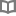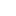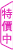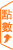哈克流浪記 - 馬克．吐溫 - BOOKWALKER中文電子書

哈克流浪記

馬克．吐溫

馬克．吐溫（Mark Twain）1835 ~ 1910馬克．吐溫Mark Twain(1835～1910)是美國十九世紀現實主義文學的代表作家。二十歲時成為密西西比河上的領航員，航運生涯經驗非常豐富，讓馬克．吐溫文思泉湧，其中《哈克歷險記》即是以密西西比河為背景的刺激探險小說。其他不朽的著作尚有《湯姆歷險記》、《鍍金時代》、《艱苦歲月》、《乞丐王子》……等。

• 放入購物車
•試閱

• 放入購物車
•試閱

• 放入購物車
•試閱

• 放入購物車
•試閱

• 放入購物車
•試閱

• 放入購物車
•試閱

• 放入購物車
•試閱

• 放入購物車
•試閱

• 放入購物車
•試閱

• 放入購物車
•無試閱

• 放入購物車
•試閱

• 放入購物車
•試閱

• 放入購物車
•試閱

• 放入購物車
•試閱

• 放入購物車
•試閱

• 放入購物車
•試閱

• 放入購物車
•試閱

• 放入購物車
•試閱

• 放入購物車
•試閱

• 放入購物車
•試閱

• 放入購物車
•試閱

• 放入購物車
•試閱• 放入購物車
•試閱

• 放入購物車
•無試閱

• 放入購物車
•試閱

• 放入購物車
•試閱• 放入購物車
•試閱• 放入購物車
•試閱

• 放入購物車
•試閱

• 放入購物車
•試閱

• 放入購物車
•試閱

• 放入購物車
•試閱

• 放入購物車
•試閱• 放入購物車
•試閱

• 放入購物車
•試閱

• 放入購物車
•試閱

• 放入購物車
•無試閱

• 放入購物車
•試閱

• 放入購物車
•試閱

• 放入購物車
•試閱

• 放入購物車
•試閱

• 放入購物車
•試閱